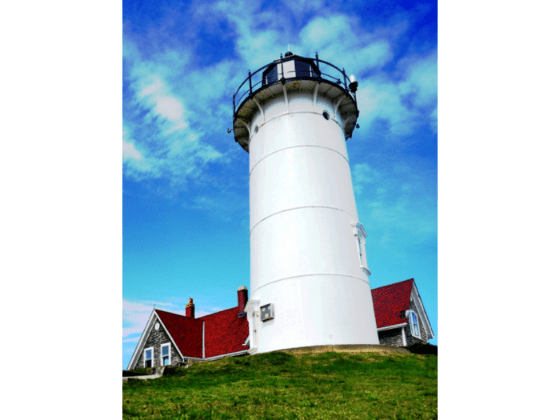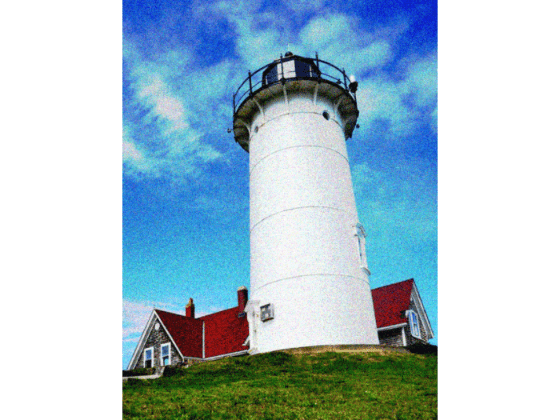# Remove Noise from Color Image Using Pretrained Neural Network

This example shows how to remove Gaussian noise from an RGB image using a denoising convolutional neural network.

Read a color image into the workspace and convert the data to data type `double`. Display the pristine color image.

```pristineRGB = imread("lighthouse.png"); pristineRGB = im2double(pristineRGB); imshow(pristineRGB) title("Pristine Image")```Add zero-mean Gaussian white noise with a variance of 0.01 to the image. The `imnoise` function adds noise to each color channel independently. Display the noisy color image.

```noisyRGB = imnoise(pristineRGB,"gaussian",0,0.01); imshow(noisyRGB) title("Noisy Image")```The pretrained denoising convolutional neural network, DnCNN, operates on single-channel images. Split the noisy RGB image into its three individual color channels.

`[noisyR,noisyG,noisyB] = imsplit(noisyRGB);`

`net = denoisingNetwork("dncnn");`

Use the DnCNN network to remove noise from each color channel.

```denoisedR = denoiseImage(noisyR,net); denoisedG = denoiseImage(noisyG,net); denoisedB = denoiseImage(noisyB,net);```

Recombine the denoised color channels to form the denoised RGB image. Display the denoised color image.

```denoisedRGB = cat(3,denoisedR,denoisedG,denoisedB); imshow(denoisedRGB) title("Denoised Image")```Calculate the peak signal-to-noise ratio (PSNR) for the noisy and denoised images. A larger PSNR indicates that noise has a smaller relative signal, and is associated with higher image quality.

```noisyPSNR = psnr(noisyRGB,pristineRGB); fprintf("\n The PSNR value of the noisy image is %0.4f.",noisyPSNR);```
``` The PSNR value of the noisy image is 20.6395. ```
```denoisedPSNR = psnr(denoisedRGB,pristineRGB); fprintf("\n The PSNR value of the denoised image is %0.4f.",denoisedPSNR);```
``` The PSNR value of the denoised image is 29.6857. ```

Calculate the structural similarity (SSIM) index for the noisy and denoised images. An SSIM index close to 1 indicates good agreement with the reference image, and higher image quality.

```noisySSIM = ssim(noisyRGB,pristineRGB); fprintf("\n The SSIM value of the noisy image is %0.4f.",noisySSIM);```
``` The SSIM value of the noisy image is 0.7393. ```
```denoisedSSIM = ssim(denoisedRGB,pristineRGB); fprintf("\n The SSIM value of the denoised image is %0.4f.",denoisedSSIM);```
``` The SSIM value of the denoised image is 0.9507. ```

In practice, image color channels frequently have correlated noise. To remove correlated image noise, first convert the RGB image to a color space with a luminance channel, such as the L*a*b* color space. Remove noise on the luminance channel only, then convert the denoised image back to the RGB color space.